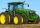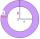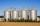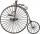Determine the radius of the circle, if its perimeter and area is the same number.

Result

r =  2

Solution:Leave us a comment of this math problem and its solution (i.e. if it is still somewhat unclear...):Be the first to comment!Next similar math problems:

1. Four circles1) Calculate the circle radius if its area is 400 cm square 2) Calculate the radius of the circle whose circumference is 400 cm. 3) Calculate circle circumference if its area is 400 cm square 4) Calculate the circle's area if perimeter 400 cm.
2. WheelsSmall tractor wheel with a diameter of 60 cm must be rotated 8 times to overcome some pathway. How many times must turn the big tractor wheel with a radius 60 cm to overcome the same distance?
3. CircleWhat is the radius of the circle whose perimeter is 6 cm?
4. Circle r,DCalculate the diameter and radius of the circle if it has length 52.45 cm.
5. AnnulusTwo concentric circles form an annulus of width 10 cm. The radius of the smaller circle is 20 cm. Calculate the content area of annulus.
6. Circle - simpleCalculate the area of a circle in dm2, if its circumference is 31.4 cm.
7. SiloOuter perimeter of silo is 32 m. Concrete wall is 35 cm thick. What is diameter and area of inside floor of silo?
8. Area to perimeterCalculate circle circumference if its area is 254.34cm2
9. MineWheel in traction tower has a diameter 5 m. How many meters will perform an elevator cabin if wheel rotates in the same direction 49 times?
10. FlowerbedIn the park there is a large circular flowerbed with a diameter of 12 m. Jakub circulated him ten times and the smaller Vojtoseven times. How many meters each went by and how many meters did Jakub run more than Vojta?
11. WheelDiameter of motocycle wheel is 52 cm. How many times rotates wheel on roand long 2 km?
12. WellRope with a bucket is fixed on the shaft with the wheel. The shaft has a diameter 50 cm. How many meters will drop bucket when the wheels turn 15 times?
13. ThalesCalculate the length of the Thales' circle described to right triangle with hypotenuse 18.4 cm.
14. Circular lawnAround a circular lawn area is 2 m wide sidewalk. The outer edge of the sidewalk is curb whose width is 2 m. Curbstone and the inner side of the sidewalk together form a concentric circles. Calculate the area of the circular lawn and the result round to 1
15. AceThe length of segment AB is 24 cm and the point M and N divided it into thirds. Calculate the circumference and area of this shape.
16. CableCable consists of 8 strands, each strand consists of 12 wires with diameter d = 0.5 mm. Calculate the cross-section of the cable.
17. Right triangle ABCCalculate the perimeter and area of a right triangle ABC, if you know the length of legs 4 cm 5.5 cm and 6.8 cm is hypotenuse.